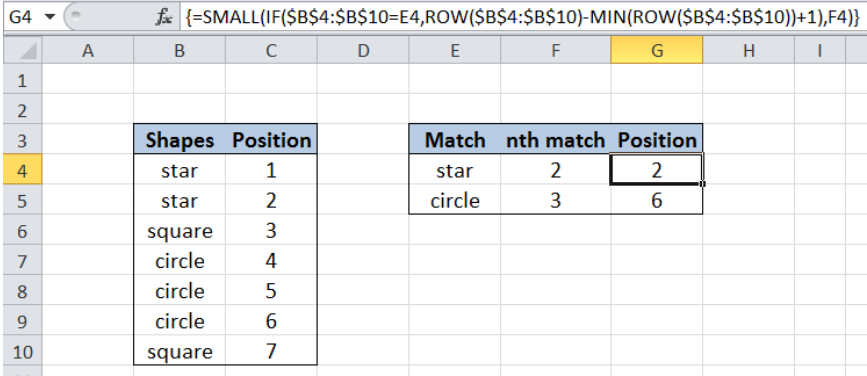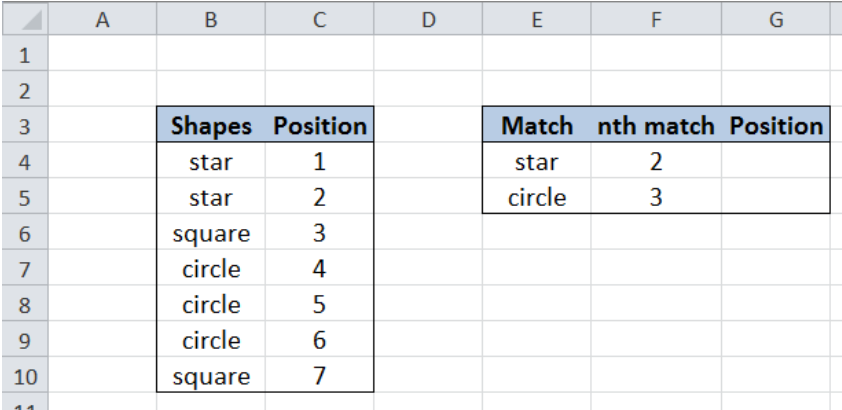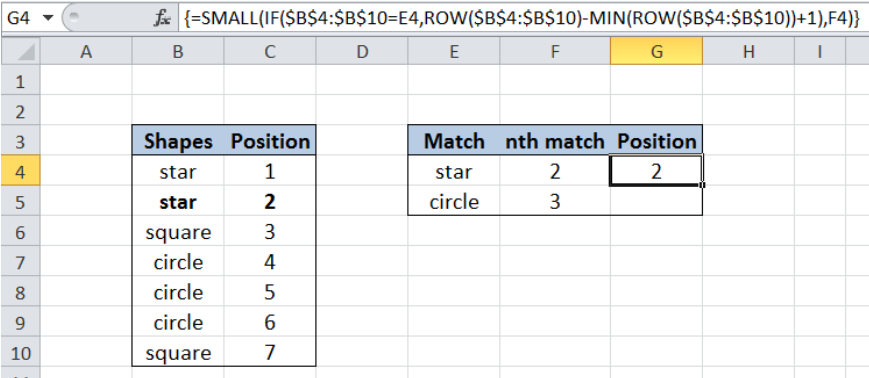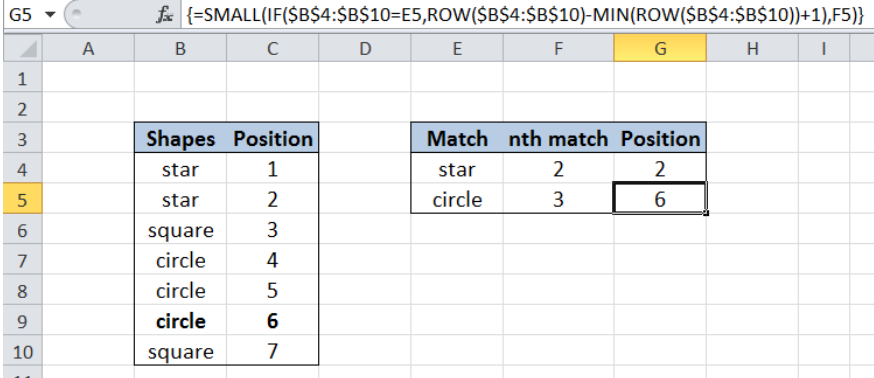Get instant live expert help with Excel or Google Sheets“My Excelchat expert helped me in less than 20 minutes, saving me what would have been 5 hours of work!”

#### Post your problem and you'll get expert help in seconds

Your message must be at least 40 characters
Our professional experts are available now. Your privacy is guaranteed.

# Get nth match

While working with Excel, we are able to match specific values in a list and find the exact position of the value by using the SMALL, IF, ROW and MIN functions.  This step by step tutorial will assist all levels of Excel users in getting the nth match of a given value.Figure 1. Final result: Get nth match

Final formula:  `=SMALL(IF(\$B\$4:\$B\$10=E4,ROW(\$B\$4:\$B\$10)-MIN(ROW(\$B\$4:\$B\$10))+1),F4)`

## Syntax of the SMALL function

SMALL returns the k-th smallest value in a data set

`=SMALL(array, k)`

• array – An array or range for which we want to determine the k-th smallest value
• k  – the position of the value in the range or data set that we want to return
• SMALL returns the #NUM! error value when the array is empty, if k≤ 0,or if k exceeds the number of data points

## Syntax of IF Function

IF function evaluates a given logical test and returns a TRUE or a FALSE

`=IF(logical_test, [value_if_true], [value_if_false])`

• The arguments “value_if_true” and “value_if_false” are optional.  If left blank, the function will return TRUE if the logical test is met, and FALSE if otherwise.

## Syntax of the ROW function

ROW function returns the row number of a reference

`=ROW(reference)`

• reference – The cell or range of cells whose row number we want to determine

## Syntax of the MIN function

MIN function returns the lowest value in a data set

`=MIN(number1, [number2], ...)  `

The parameters are:

• number1, number2, … – the numbers for which we want to find the lowest value; only number1 is required; succeeding numbers are optional
• The arguments could be numbers, array or reference to cells containing numbers

## Setting up Our DataFigure 2. Sample data to get nth match

Our table contains two columns: Shapes (column B) and Position (column C).  We included column C here for better understanding and for easy reference. In column E, we enter the shapes that we want to match while in column F, we enter the nth match we want to find for the specific shapes.  In column G, we want to determine the position of the 2nd match for star and the third match for circle.

## Get position of 2nd match of star

In order to determine the position of the 2nd match for star, we follow these steps:

Step 1.  Select cell G4

Step 2.  Enter the array formula: `=SMALL(IF(\$B\$4:\$B\$10=E4,ROW(\$B\$4:\$B\$10)-MIN(ROW(\$B\$4:\$B\$10))+1),F4)`

Step 3:  Select G4 and press Ctrl + Shift + Enter to activate the array formula

The dollar signs “\$” in the formula fix the cells so that we can easily copy and paste the formula to other cells.Figure 3.  Getting the 2nd match for star

Our array for the SMALL function is the IF function, while the value for k is F4 or 2.  This means that we want to find the 2nd smallest value from the array resulting from the IF function.

The IF function has the condition `\$B\$4:\$B\$10=E4` which means that we want to find only the values in column B that are equal to E4 or star.

The logical test returns the array: `{``TRUE;TRUE``;FALSE;FALSE;FALSE;FALSE;FALSE}` because “star” is positioned 1st and 2nd in the list.

If the logical test returns true, the value returned is determined by the ROW and MIN functions:

`ROW(\$B\$4:\$B\$10)-MIN(ROW(\$B\$4:\$B\$10))+1)`

• The ROW function returns an array of the row numbers of B4:B10: `{4;5;6;7;8;9;10}`.
• The MIN function returns the smallest row number of the range B4:B10, which is 4.
• The formula becomes: `{4;5;6;7;8;9;10} - 4 +1.= ``{1;2;3;4;5;6;7}`

Applying the IF function to the array formula results to

` {1;2``;FALSE;FALSE;FALSE;FALSE;FALSE}` because only the first two values in the list is equal to “star”.

Finally, the SMALL function returns the 2nd smallest value in the array, so the result in cell G4 is 2, which is the position of the 2nd match of “star”.

## Get position of 3rd match of circle

In order to determine the position of the 3rd match for circle, we follow these steps:

Step 1.  Copy the formula in cell G4 to cell G5 by clicking the “+” icon at the bottom-right corner of cell G4 and dragging it down.

Step 2.   Select cell G5 and press Ctrl + Shift + Enter to activate the array formula.Figure 4. Getting the 3rd match for circle

Most of the time, the problem you will need to solve will be more complex than a simple application of a formula or function. If you want to save hours of research and frustration, try our live Excelchat service! Our Excel Experts are available 24/7 to answer any Excel question you may have. We guarantee a connection within 30 seconds and a customized solution within 20 minutes.

### Did this post not answer your question? Get a solution from connecting with the expert.Another blog reader asked this question today on Excelchat:## Subscribe to Excelchat.coAnother blog reader asked this question today on Excelchat: